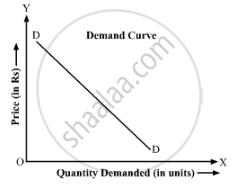Share

# Give Reason Or Explain the Following Statement. Demand Curve Slopes Downward from Left to Right. - Economics

ConceptDemand

#### Question

Give reason or explain the following statement.

Demand curve slopes downward from left to right.

#### Solution

Demand curve is the graphical representation of the relationship between the demand for a good and its price, for a given income, price of related goods, tastes and preferences. This curve slopes downwards from left to right because of the negative relationship between price of the commodity and its demand.Is there an error in this question or solution?

#### APPEARS IN

Micheal Vaz Solution for Micheal Vaz Class 12 Economics (2019 to Current)
Chapter 3: Demand Analysis
Exercise | Q: 2.3 | Page no. 24

#### Video TutorialsVIEW ALL 

Solution Give Reason Or Explain the Following Statement. Demand Curve Slopes Downward from Left to Right. Concept: Demand.
S